Next: Desargues Theorem Up: Hyperplanes and Duality Previous: Hyperplanes and Duality

### The Duality Principle

The set of all points in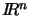whose coordinates satisfy a linear equation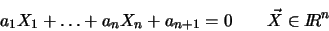is called a hyperplane. Substituting homogeneous coordinates Xi = xi/xn + 1 and multiplying out, we get a homogeneous linear equation that represents a hyperplane in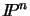: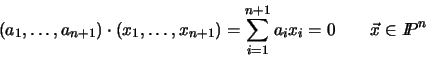(2.2)

Notice the symmetry of equation (2.2) between the hyperplane coefficients (a1, ..., an + 1) and the point coefficients (x1, ..., xn + 1). For fixed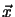and variable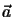, (2.2) can also be viewed as the equation characterizing the hyperplanespassing through a given point. In fact, the hyperplane coefficientsare also only defined up to an overall scale factor, so the space of all hyperplanes can be considered to be another projective space called the dual of the original space. By the symmetry of (2.2), the dual of the dual is the original space.

An extremely important duality principle follows from this symmetry:

Duality Principle: For any projective result established using points and hyperplanes, a symmetrical result holds in which the roles of hyperplanes and points are interchanged: points become planes, the points in a plane become the planes through a point, etc.

For example, in the projective plane, any two distinct points define a line (i.e. a hyperplane in 2D). Dually, any two distinct lines define a point (their intersection). Note that duality only holds universally in projective spaces: for example in the affine plane parallel lines do not intersect at all.Next: Desargues Theorem Up: Hyperplanes and Duality Previous: Hyperplanes and Duality
Bill Triggs
1998-11-13